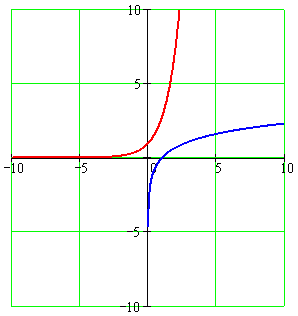Calculus on Demand at Dartmouth College Lecture 3 | Index | Lecture 5 Lecture 4Resources

Math 3 Course Syllabus
Practice Exams

Contents

In this lecture we will discuss exponential and logarithmic functions, and their graphs. These functions turn out be very useful in modeling growth of populations. They are central to formulas for compound interest in banking and in computing ages of objects using radioactive decay.

Quick Question

What is the relationship between the graphs of ex and ln(x)?Outline

Outline for Exponential and Logarithmic Functions

Textbook

Exponential and Logarithmic Functions